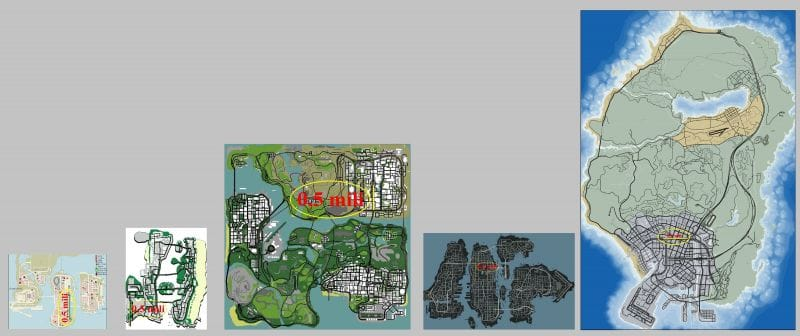# GTA: Ranking the maps in order of sizeMaps have always been an intrinsic part of the highly popular GTA series as the vastness of the open world that the games offer is reflected in them.

The size of every map in the GTA games is different. A member of the GTAForums, named KeWiS, has done a phenomenal job in determining the size of the maps in the series. He has taken into consideration three types of areas while calculating the size of the GTA maps. The three areas are Total Area, Water Area and Land Area.

Here are all the maps in the GTA series ranked based on their size:## Maps of GTA games ranked in order of sizeSize of the maps on the basis of Land Area (Image Courtesy: GTAForums)

Based on the findings of KeWiS, the sizes of the following maps are presented based on their respective area types.

### Map size based on Land Area

#5 GTA 3 = 4.38 square kilometers.

#4 GTA: Vice City = 5.62 square kilometres.

#3 GTA 4 =8.06 square kilometres.

#2 GTA: San Andreas = 31.55 square kilometres.

#1 GTA 5 = 48.15 square kilometers.

### Map size based on Water Area

#5 GTA 3 = 3.76 square kilometers.

#4 GTA: Vice City = 3.5 square kilometres.

#3 GTA 4 = 8.08 square kilometers.

#2 GTA: San Andreas = 6.67 square kilometres.

#1 GTA 5 = 27.69 square kilometers.

### Map size based on Total Area

#5 GTA 3 = 8.12 square kilometers.

#4 GTA: Vice City = 9.11 square kilometres.

#3 GTA 4 = 16.14 square kilometers.

#2 GTA: San Andreas = 38.2 square kilometres.

#1 GTA 5 = 75.84 square kilometers.

Based on the above measurements, we can conclude that GTA 5 is the biggest map in the series and GTA 3 is the smallest.

Edited by Rachel Syiemlieh
Be the first one to commentGIFReplyGIF